# KSEEB Solutions for Class 6 Maths Chapter 14 Practical Geometry Ex 14.5

Students can Download Chapter 14 Practical Geometry Ex 14.5 Questions and Answers, Notes Pdf, KSEEB Solutions for Class 6 Maths helps you to revise the complete Karnataka State Board Syllabus and score more marks in your examinations.

## Karnataka State Syllabus Class 6 Maths Chapter 14 Practical Geometry Ex 14.5

Question 1.
Draw $$\overline{\mathbf{A B}}$$ of length 7.3 cm and find its axis of symmetry.
Solution:
The below given steps will be followed to construct AB of length 7.3 cm and to find its axis of symmetry.
(1) Draw a line segment $$\overline{\mathbf{A B}}$$ of 7.3 cm.
(2) Taking A as centre, draw a circle by using compasses. The radius of circle should be more than half the length of $$\overline{\mathbf{A B}}$$.
(3) With the same radius as before, draw another circle using compasses while taking point B as centre. Let it cut the previous circle at C and D.
(4) Join $$\overline{\mathbf{C D}}$$. $$\overline{\mathbf{C D}}$$ is the axis of symmetry.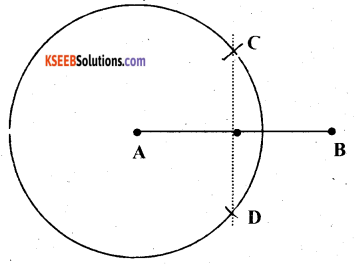Question 2.
Draw a line segment of length 9.5 cm and construct its perpendicular bisector.
Solution: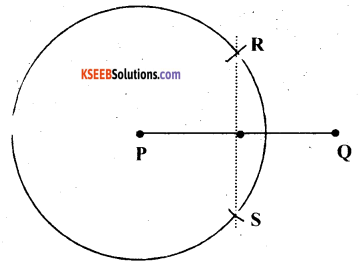The below given steps will be followed to construct a line segment of length 9.5 cm and its perpendicular bisector.
(1) Draw a line segment $$\overline{\mathrm{PQ}}$$ of 9.5 cm.
(2) Taking P as centre, draw a circle by using compasses. The radius of circle should be more than half the length of $$\overline{\mathrm{PQ}}$$.
(3) With the same radius as before, draw another circle using compasses while taking point Q as centre. Let it cut the previous circle at R and S.
(4) Join RS. $$\overline{\mathrm{RS}}$$ is the axis of symmetry i.e., the perpendicular bisector of line PQ.Question 3.
Draw the perpendicular bisector of $$\overline{\mathrm{XY}}$$ whose length is 10.3 cm.
(a) Take any point P on the bisector drawn. Examine whether PX = PY.
(b) If M is the mid point of $$\overline{\mathrm{XY}}$$, what can you say about the lengths MX and XY?
Solution: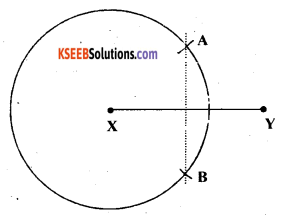(1) Draw a line segment $$\overline{\mathrm{XY}}$$ of 10.3 cm.
(2) Taking point X as centre, draw.a circle by using compasses. The radius of circle should be more than half the length of $$\overline{\mathrm{XY}}$$.
(3) With the same radius as before, draw another circle using compasses while taking point Y as centre. Let it cut the previous circle at A and B.
(4) Join $$\overline{\mathrm{AB}}$$ $$\overline{\mathrm{AB}}$$ is the axis of symmetry.
(a) Take any point P on $$\overline{\mathrm{AB}}$$. We will find that the measures of the lengths of PX and PY are same.
It is because $$\overline{\mathrm{AB}}$$ the axis of symmetry. Hence, any point lying on $$\overline{\mathrm{AB}}$$ will be at the same distance from both the ends of $$\overline{\mathrm{XY}}$$.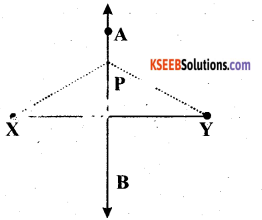(b) M is the mid-point of $$\overline{\mathrm{XY}}$$. Perpendicular bisector $$\overline{\mathrm{AB}}$$ will be passing through point M. Hence, length of $$\overline{\mathrm{XY}}$$ is just double of $$\overline{\mathrm{MX}}$$ Or, 2MX = XY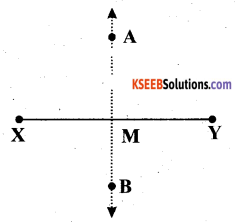Question 4.
Draw a line segment of length 12.8 cm. Using compasses, divide it into four equal parts. Verify by actual measurement.
Solution: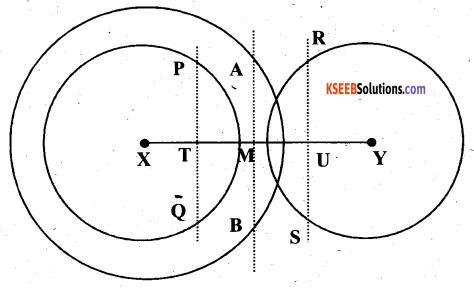(1) Draw a line segment $$\overline{\mathrm{XY}}$$ of 12.8 cm.
(2) Draw a circle, while taking point X as centre and radius more than half of XY.
(3) With same radius and taking centre as Y, again draw arcs to cut the circle at Aand B. Join AB which intersects $$\overline{\mathrm{XY}}$$ at M.
(4) Taking X and Y as centres, draw two circles with radius more than half of $$\overline{\mathrm{XM}}$$.
(5) With same radius and taking M as centre, draw arcs to intersect these circles at P, Q and R, S.
(6) Join PQ and RS. These are intersecting $$\overline{\mathrm{XY}}$$ at T and U.
(7) Now, $$\overline{\mathrm{XT}}$$ = $$\overline{\mathrm{TM}}$$ = $$\overline{\mathrm{MU}}$$ = $$\overline{\mathrm{UY}}$$. These are 4 equal parts of $$\overline{\mathrm{XY}}$$.
By measuring these line segments with the help of ruler, we will find that each is of 3.2 cm.Question 5.
With $$\overline{\mathrm{PQ}}$$ of length 6.1 cm as diameter, draw a circle.
Solution: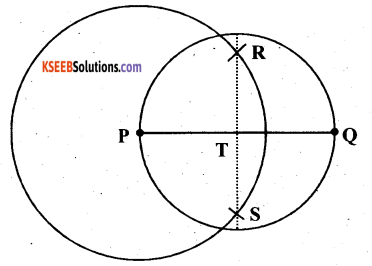(1) Draw a line segment of 6.1 cm.
(2) Taking point P as centre and radius more than half of $$\overline{\mathrm{PQ}}$$, draw a circle.
(3) With same radius,and taking Q as centre, draw arcs to intersect this circle at points R and S.
(4) Join RS which intersects $$\overline{\mathrm{PQ}}$$ at T.
(5) Taking T as centre and with radius TP, draw a circle which will also pass through Q. It is the required circle.

Question 6.
Draw a circle with centre C and radius 3.4 cm. Draw any chord $$\overline{\mathrm{AB}}$$. Construct the perpendicular bisector of $$\overline{\mathrm{AB}}$$ and examine if it passes through C.
Solution: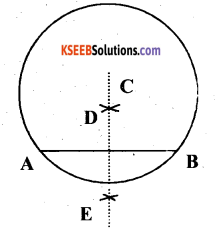(1) Mark any point Con the sheet.
(2) By adjusting the compasses up to 3.4 cm and by putting the pointer of the compasses at point C, turn the compasses slowly to draw the .circle. It is the required circle of 3.4 cm radius.
(3) Now, mark any chord $$\overline{\mathrm{AB}}$$ in the circle.
(4) Taking A and B as centres, draw arcs on both sides of $$\overline{\mathrm{AB}}$$. Let these intersect each other at D and E.
(5) Join DE, which is the perpendicular bisector of AB. When $$\overline{\mathrm{DE}}$$ is extended, it will pass through point C.Question 7.
Draw a circle with centre C and radius 3.4 cm. Draw any chord $$\overline{\mathrm{AB}}$$. Construct the perpendicular bisector of $$\overline{\mathrm{AB}}$$ and examine if it passes through C, if $$\overline{\mathrm{AB}}$$ happens to be a diameter.
Solution:
(1) Mark any point C on the sheet.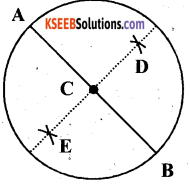(2) By adjusting the compasses up to 3.4 cm and by putting the pointer of the compasses at point C, turn the compasses slowly to draw the circle. It is the required circle of 3 .4 cm radius.
(3) Mark any diameter $$\overline{\mathrm{AB}}$$ in the circle.
(4) Now, taking Aand B as centres, draw arcs on both sides of $$\overline{\mathrm{AB}}$$ taking radius more than $$\overline{\mathrm{AB}}$$. Let these intersect each other at D and E.
(5) Join DE, which is the perpendicular bisector of AB. It can be observed that $$\overline{\mathrm{DE}}$$ is passing through the centre C of the circle.

Question 8.
Draw a circle of radius 4 cm. Draw any two of its chords. Construct the perpendicular bisectors of these chords. Where do they meet?
Solution:
1) Mark any point C on the sheet. Now, by adjusting the compasses up to 4 cm and by putting the pointer of compasses at point C, turn the compasses slowly to draw the circle. It is the required circle of 4 cm radius.
(2) Take any two chords $$\overline{\mathrm{AB}}$$ and $$\overline{\mathrm{CD}}$$ in the circle.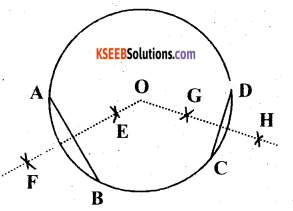(3) Taking A and B as centres and with radius more than half of $$\overline{\mathrm{AB}}$$, draw arcs on both sides of AB, intersecting each other at E, F. Join EF which is the perpendicular bisector of AB.
(4) Taking C and D as centres and with radius more than half of $$\overline{\mathrm{CD}}$$, draw arcs o n both sides of CD, intersecting each other at G, H. Join GH which is the perpendicular bisector of CD. Now, we will find that when EF and GH are extended, they meet at the centre of the circle i.e., point O.Question 9.
Draw any angle with vertex O. Take a point A on one of its arms and B on another such that OA = OB. Draw the perpendicular bisectors of $$\overline{\mathbf{O A}}$$ and $$\overline{\mathbf{O B}}$$. Let them meet at P. Is PA = PB ?
Solution:
(1) Draw any angle whose vertex is O.
(2) With a convenient radius, draw arcs on both rays of this angle while taking O as centre. Let these points be A and B.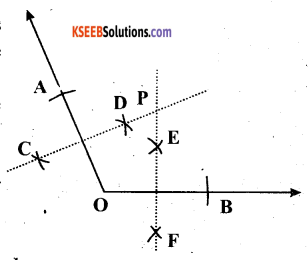(3) Taking O and A as centres and with radius more than half of OA, draw arcs on both sides of OA. Let these be intersecting at C and D. Join CD.
(4) Similarly, we can find the perpendicular bisector $$\overline{\mathbf{E F}}$$ and $$\overline{\mathbf{O B}}$$. These perpendicular bisectors $$\overline{\mathbf{C D}}$$ and $$\overline{\mathbf{E F}}$$ will intersect each other at P.
Now, PA and PB can be measured. These are equal in length.